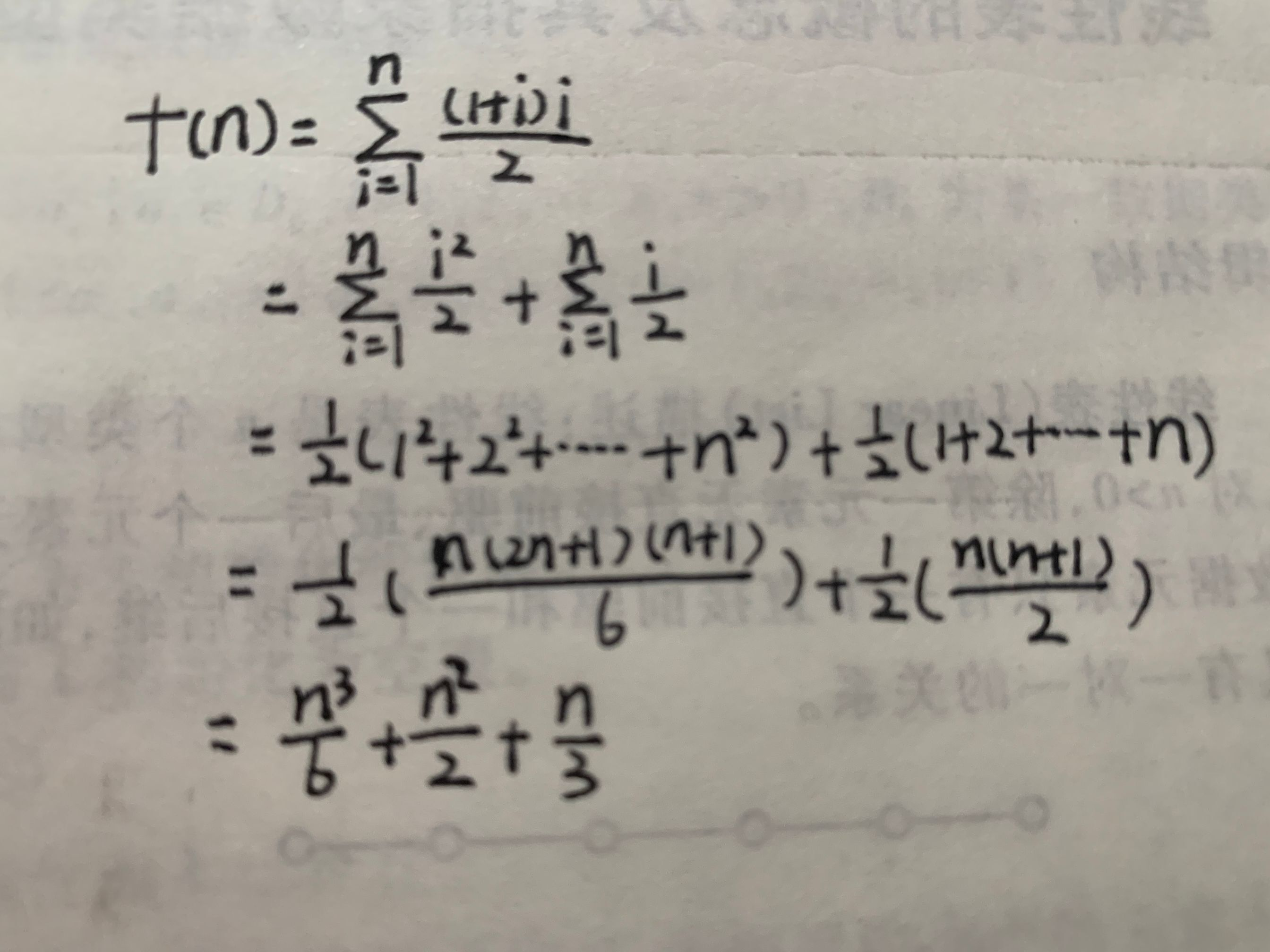# 数据结构---三重循环的语句频度

## 题目

for( i = 1 ; i <= n ; i++ )
for( j = 1 ; j <= i ; j++ )
for( k = 1 ; k <= j ; k++)
x = x + 1;


(出自：《数据结构——用C语言描述》(第二版) 耿国华著）

for( i = 1 ; i <= n ; i++ )				//设为语句1
for( j = 1 ; j <= i ; j++ )			//设为语句2
S;


for( j = 1;j <= i; j++)
for( k =1;k <=j;k++)
x = x + 1;f(n) = (n+2)*(n+1)*n/6

03-28470106-30969
04-281238
11-136487
08-17103
09-061856
04-1735
03-23223
09-15446
12-0352
03-142118
01-052万+
12-20121
09-1068
08-1384
09-10169
06-21385
09-28896